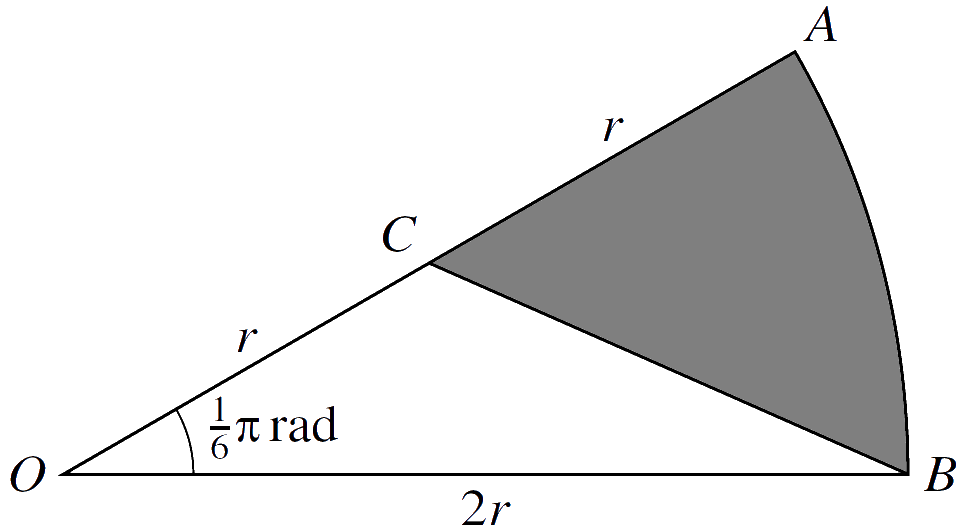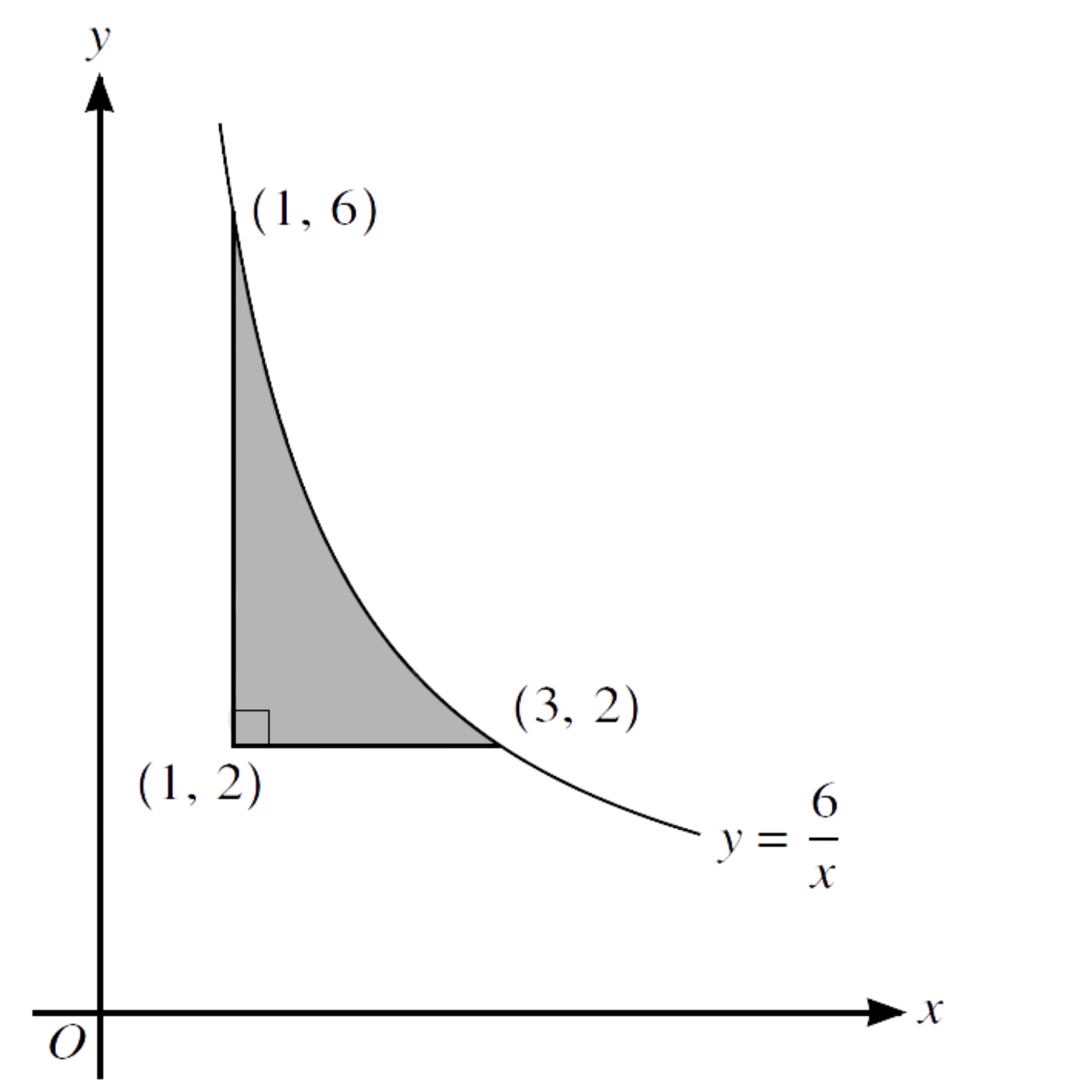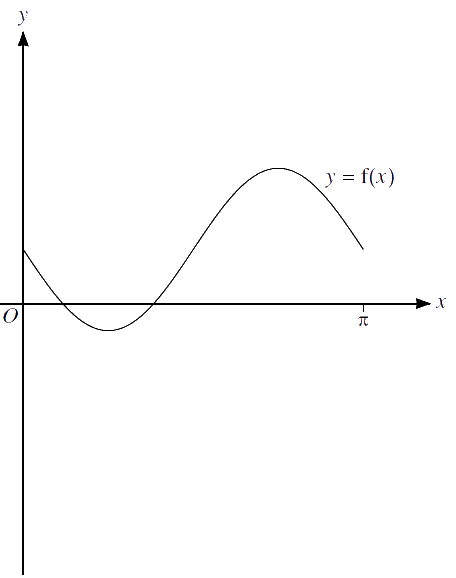Home » 9709 » Paper 12 May June 2020 Pure Math I – 9709/12/M/J/20

Check out my complete solution here:
» Full Solutions «
Like and subscribe too! =)

1.     (a)     Find the coefficient of $\ x^2 \$ in the expansion of $\ {(x \ – \ \frac{2}{x})}^{6}$.
$$\tag*{}$$
(b)     Find the coefficient of $\ x^2 \$ in the expansion of $\ (2 + 3{x}^{2}){(x \ – \ \frac{2}{x})}^{6}$.
$$\tag*{}$$

2.     (a)     Express the equation $\ 3 \cos \theta = 8 \tan \theta \$ as a quadratic equation in $\ \sin \theta$.
$$\tag*{}$$
(b)     Hence find the acute angle, in degrees, for which $\ 3 \cos \theta = 8 \tan \theta$.
$$\tag*{}$$

3.     A weather balloon in the shape of a sphere is being inflated by a pump. The volume of the balloon is increasing at a constant rate of $\ 600 \ {\mathrm{cm}}^{3} \$ per second. The balloon was empty at the start of pumping.

(a)     Find the radius of the balloon after 30 seconds.
$$\tag*{}$$
(b)     Find the rate of increase of the radius after 30 seconds.
$$\tag*{}$$

4.     The $\ n$th term of an arithmetic progression is $\ \frac{1}{2}(3n \ – \ 15)$.

Find the value of $\ n \$ for which the sum of the first $\ n \$ terms is 84.
$$\tag*{}$$

5.     The function $\ \mathrm{f} \$ is defined for $\ x \in \mathbb{R} \$ by
$$\ \mathrm{f} : x \mapsto \ a \ – \ 2x,$$
where $\ a \$ is a constant.

(a)     Express $\ \mathrm{f}\mathrm{f}(x) \$ and $\ {\mathrm{f}}^{-1}(x) \$ in terms of $\ a \$ and $\ x$.
$$\tag*{}$$
(b)     Given that $\ \mathrm{f}\mathrm{f}(x) = {\mathrm{f}}^{-1}(x)$, find $\ x \$ in terms of $\ a$.
$$\tag*{}$$

6.     The equation of a curve is $\ y = 2x^2 + kx + k \ − \ 1$, where $\ k \$ is a constant.

(a)     Given that the line $\ y = 2x + 3 \$ is a tangent to the curve, find the value of $\ k$.
$$\tag*{}$$

It is now given that $\ k = 2$.

(b)     Express the equation of the curve in the form $\ y = 2{(x + a)}^{2} + b$, where $\ a \$ and $\ b \$ are constants, and hence state the coordinates of the vertex of the curve.
$$\tag*{}$$

7.In the diagram, $\ OAB \$ is a sector of a circle with centre $\ O \$ and radius $\ 2r$, and angle $\ AOB = \frac{1}{6} \pi \$ radians. The point $\ C \$ is the midpoint of $\ OA$.

(a)     Show that the exact length of $\ BC \$ is $\ r \sqrt{5 \ – \ 2 \sqrt{3}}$.
$$\tag*{}$$
(b)     Find the exact perimeter of the shaded region.
$$\tag*{}$$
(c)     Find the exact area of the shaded region.
$$\tag*{}$$

8.(a)     Find the volume generated when the shaded region is rotated through $\ {360}^{\circ} \$ about the $\ \textbf{y-axis}$.
$$\tag*{}$$
(b)     The tangent to the curve at a point $\ X \$ is parallel to the line $\ y + 2x = 0$. Show that $\ X \$ lies on the line $\ y = 2x$.
$$\tag*{}$$

9.     Functions $\ \mathrm{f} \$ and $\ \mathrm{g} \$ are such that
$$\mathrm{f}(x) = 2 \ – \ 3 \sin 2x \enspace \textrm{for} \ 0 \le x \le \pi,$$
$$\mathrm{g}(x) = -2 \mathrm{f}(x) \enspace \textrm{for} \ 0 \le x \le \pi.$$
(a)     State the ranges of $\ \mathrm{f} \$ and $\ \mathrm{g}$.
$$\tag*{}$$
The diagram below shows the graph of $\ y = f(x)$.(b)     Sketch, on this diagram, the graph of $\ y = g(x)$.
$$\tag*{}$$
The function $\ \mathrm{h} \$ is such that
$$\mathrm{h}(x) = \mathrm{g}(x+\pi) \enspace \textrm{for} \ -\pi \le x \le 0.$$
(c)     Describe fully a sequence of transformations that maps the curve $\ y = f(x) \$ on to $\ y = h(x)$.
$$\tag*{}$$

10.     The equation of a curve is $\ y = 54x \ − \ {(2x − 7)}^{3}$.

(a)     Find $\ \frac{\mathrm{d}y}{\mathrm{d}x} \$ and $\ \frac{{\mathrm{d}}^{2}y}{{\mathrm{d}}^{2}x}$.
$$\tag*{}$$
(b)     Find the coordinates of each of the stationary points on the curve.
$$\tag*{}$$
(c)     Determine the nature of each of the stationary points.
$$\tag*{}$$

11.     The equation of a circle with centre $\ C \$ is $\ x^2 + y^2 − 8x + 4y − 5 = 0$.

(a)     Find the radius of the circle and the coordinates of $\ C$.
$$\tag*{}$$
The point $\ P(1, 2) \$ lies on the circle.

(b)     Show that the equation of the tangent to the circle at $\ P \$ is $\ 4y = 3x + 5$.
$$\tag*{}$$
The point $\ Q \$ also lies on the circle and $\ PQ \$ is parallel to the $\ x$-axis.

(c)     Write down the coordinates of $\ Q$.
$$\tag*{}$$
The tangents to the circle at $\ P \$ and $\ Q \$ meet at $\ T$.

(d)     Find the coordinates of $\ T$.
$$\tag*{}$$